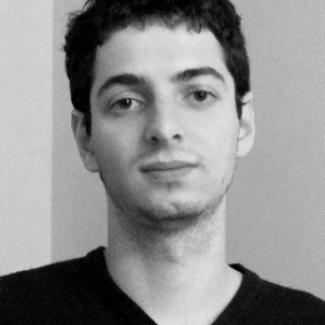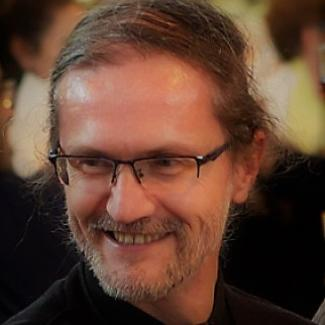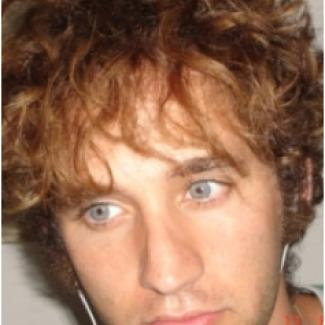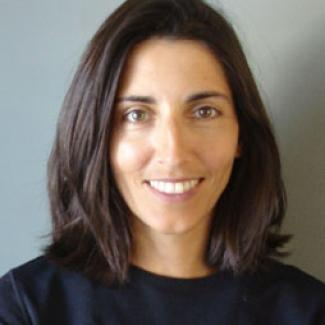Meta-complexity refers to the complexity of computational problems and tasks that are themselves about computations and their complexity. For example, the Minimum Circuit Size Problem (given the truth table of a Boolean function F, compute the minimum circuit size of F) and the problem Kpoly of determining the polynomial-time bounded Kolmogorov complexity of a string are key meta-complexity problems. Meta-complexity provides a unifying framework for a variety of important tasks in several important areas of computer science, including computational complexity, proof complexity, cryptography, and learning theory.

These areas are all intimately linked, but only recently are these links being made explicit and studied more closely. For example, learning can be interpreted as solving search versions of the Minimum Circuit Size Problem and related problems. Basing primitives such as one-way functions and indistinguishability obfuscation on standard complexity assumptions is one of the main objectives in theoretical cryptography. Important recent directions involving meta-complexity within proof complexity, such as lifting and automatability, strengthen analogies and connections between proof complexity and circuit complexity. In addition, independence results such as the natural proofs framework have intuitive interpretations in terms of meta-complexity. These connections have led to several recent breakthroughs, including quasi-polynomial time PAC-learning algorithms for constant-depth circuits with parity gates [Carmosino-Impagliazzo-Kabanets-Kolokolova16], new worst-case to average-case reductions for NP problems [Hirahara18], a new complexity-theoretic characterization of one-way functions [Liu-Pass20], and the NP-hardness of automating Resolution [Atserias-Muller19].

The program will bring together researchers in computational complexity, proof complexity, cryptography, and learning theory for a renewed attack on fundamental problems in these areas by exploiting the tools and techniques of meta-complexity. We also wish to broaden the scope of meta-complexity to areas such as the theory of total function NP search problems, algebraic complexity, and quantum complexity. Among the fundamental problems we plan to tackle are: Is the Minimum Circuit Size Problem NP-hard? Can one-way functions be based on NP-hardness? What are the minimal complexity assumptions for indistinguishability obfuscation? Are DNFs hard to PAC-learn under standard complexity assumptions? Is Resolution weakly automatable?

## OrganizersIgor Carboni Oliveira (University of Warwick)Valentine Kabanets (Simon Fraser University)Rafael Pass (Tel-Aviv University and Cornell Tech)Toni Pitassi (University of Toronto)Rahul Santhanam (University of Oxford)

## Long-Term Participants (including Organizers)

Yuval Ishai (Technion Israel Institute of Technology)